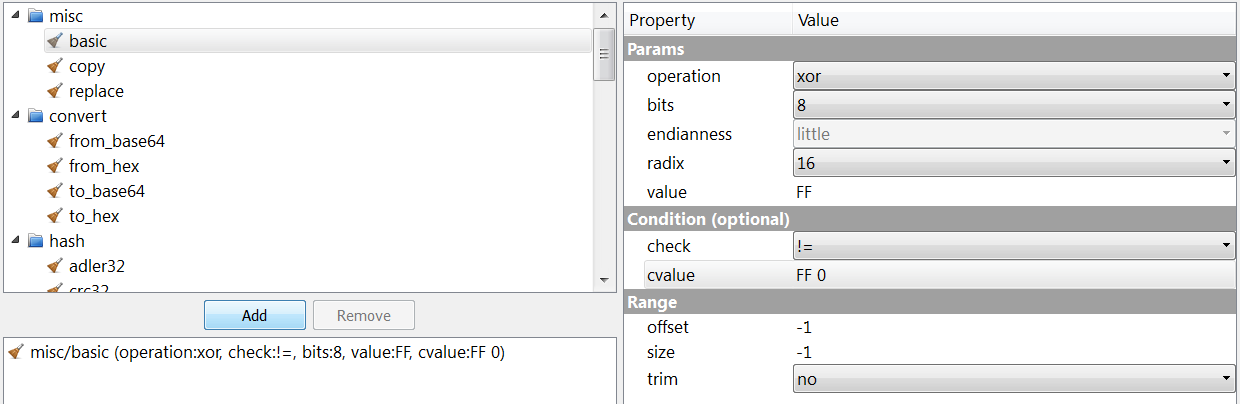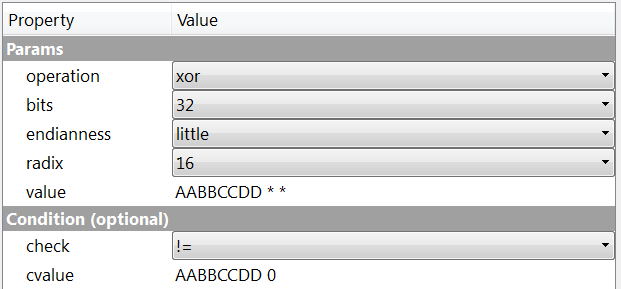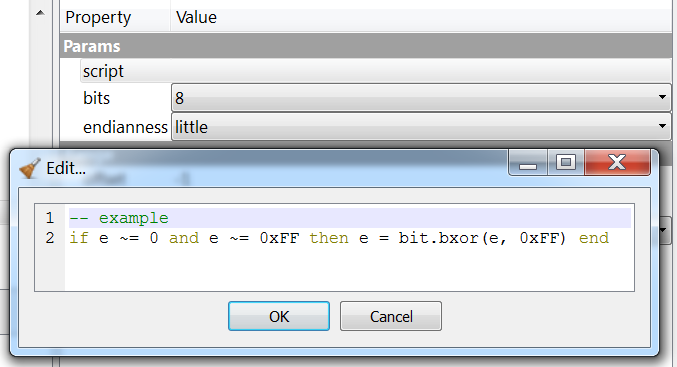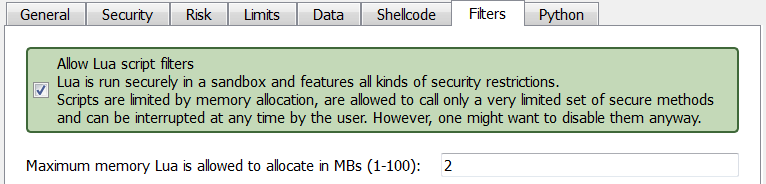# Custom filters: Lua and misc/basic

Last year filters have been introduced and among them them the very useful ‘misc/basic‘. The upcoming 0.9.5 version of the Profiler improves this filter introducing the condition parameter.

For instance, let’s take the following filter:It xors every byte if different than 0xFF and 0. The ‘misc/basic‘ filter can be used to express even more complex operations such as:In this case the the filter xors every third dword with 0xAABBCCDD, following the pattern ‘xor skip skip’, in little endian mode and only if the value is different than 0 and 0xAABBCCDD. While lots of operations can be expressed with this filter, there are limits.

This is why Lua filters have been introduced. Right now there are two such filters available: ‘lua/custom‘ and ‘lua/loop‘. Let’s start with the second one which is just a shortcut.`if e ~= 0 and e ~= 0xFF then e = bit.bxor(e, 0xFF) end`

This script does the exact same thing as the first example of the ‘misc/basic‘ filter: it xors every byte if different than 0xFF and 0. In this specific case there’s no reason to use a Lua filter. In fact, Lua filters are considerably slower than native filters. Thus, they should be used only when the operation is too complex to be expressed with any of the default filters.

While ‘lua/loop‘ is inteded for simple loop operations, ‘lua/custom‘, as the name suggests, can be used to implement a custom filter logic. Here’s an example, which again does the same thing as the previous example:

```function run(filter)
local c = filter:container()
local size = c:size()
local offset = 0
local bsize = 16384
while size ~= 0 do
if bsize > size then bsize = size end
local boffs = 0
while boffs < bsize do
if e ~= 0 and e ~= 0xFF then e = bit.bxor(e, 0xFF) end
block:writeU8(boffs, e)
boffs = boffs + 1
end
c:write(offset, block)
offset = offset + bsize
size = size - bsize
end
return Base.FilterErr_None
end```

The security of these scripting filters is very high. They run in a special sandboxed environment, have access only to a minimum set of secure functions, are limited in memory consumption (2 MBs by default, but it can be configured from the settings) and can be interrupted at any time by the user.

If you still don't wish to allow script filters, they can be disabled from the settings.The Lua VM is almost vanilla, the only difference is that it allows for 64-bit numbers. As you can observe from the examples, the Lua library for bitwise operations has been renamed from 'bit32' to 'bit'.

We'll see some practical usage samples in the near future. Stay tuned!